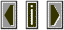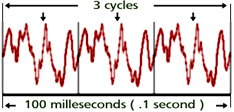FrequencyFrequency modulation in its simplest form involves The repetition rate of some periodic vibration pattern, usually described in terms of repetitions per second.If, for example, we say that a musical tone has a frequency of 300 hertz, this indicates that the waveform repeats itself 300 times each second. Frequency is inversely related period as given in the following equation:
Frequency = 1 / period
 In the example above the frequency of the signal can be determined from the two measurements given:
3 cycles / .1 second = 30 Hz
 The discrete frequencies that make up a complex timbre can be found using Fourier analysis. Frequency is correlated with the subjective sensation of pitch.